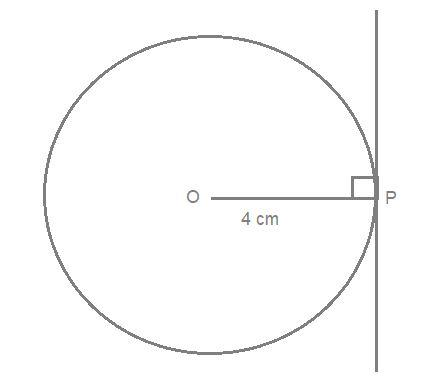QUESTION

# Draw a circle of radius 4 cm and construct a tangent at any point on the circle.

Hint: Here we go through by first drawing the circle with a given arc. Then take any point on the circle and join it with the center and now then draw a perpendicular line on that point. To make the tangent.

Here in the question it is given that the radius of the circle is 4 cm.

And we have to draw a tangent at any point on the circle.

As we know a tangent to a circle is a straight line which touches the circle at only one point. This point is called the point of tangency. The tangent to a circle is perpendicular to the radius at the point of tangency.

Now first we construct a circle

Take a measure of 4 cm by the compass on a scale and draw a circle with it. By this we made a circle with radius=4 cm.

After that we take a point P on the circle.

Now join this point with center o.

After that we draw a line perpendicular to the line OP by the help of a protector or by a compass.

The perpendicular line we draw is the required tangent to the circle.

Note: Whenever we face such a question of construction the key concept for the drawing is always make these constructions with sharp pencil and always draw circles with the help of a compass. And mark the points clearly for better understanding.